# Precalculus : Powers and Roots of Complex Numbers

## Example Questions

1 3 Next →

### Example Question #21 : Powers And Roots Of Complex Numbers

Solve for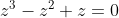(there may be more than one solution).

Possible Answers: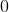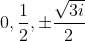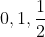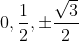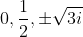Correct answer:Explanation:

To solve for the roots, just set equal to zero and solve for z using the quadratic formula, which is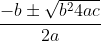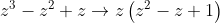and now setting both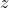and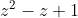equal to zero we end up with the answers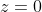and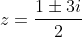and so the correct answer is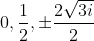.

### Example Question #11 : Find The Roots Of Complex Numbers

Solve for all possible solutions to the quadratic expression: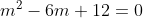Possible Answers: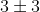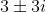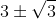Explanation:

Solve for complex values of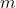using the quadratic formula: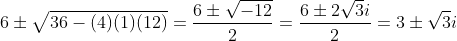.

### Example Question #23 : Powers And Roots Of Complex Numbers

Solve for(there may be more than one solution).

Possible Answers:Correct answer:Explanation:

To solve for the roots, just set equal to zero and solve forusing the quadratic formula (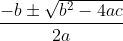):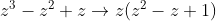and now setting bothandequal to zero we end up with the answersand.

1 3 Next →

### All Precalculus Resources